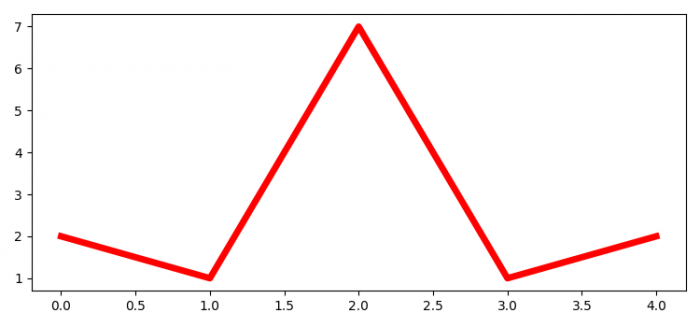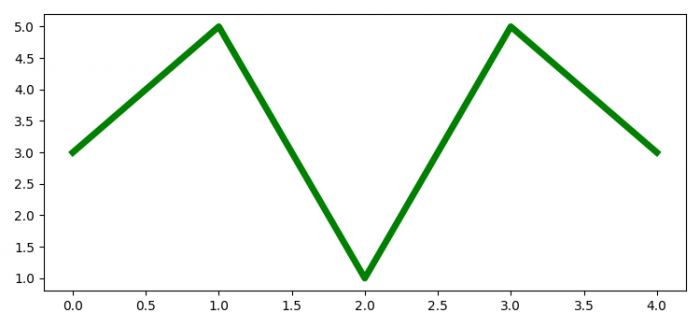# Saving all the open Matplotlib figures in one file at once

To save all the open Matplotlib figures in one file at once, we can take follwong steps −

• Set the figure size and adjust the padding between and around the subplots.
• Create a new figure (fig1) or activate an existing figure using figure() method.
• Plot the first line using plot() method.
• Create a new figure (fig2) or activate an existing figure using figure() method.
• Plot the Second line using plot() method.
• Initialize a variable, filename, to make a pdf file.
• Create a user-defind function, save_multi_image, and call it to save all the open matplotlib figures in one file at once. Create a new PdfPages object, pp.
• Get the number of open figures.
• Iterate the opened figures and save them into a file.
• To display the figure, use show() method.

## Example

from matplotlib import pyplot as plt
from matplotlib.backends.backend_pdf import PdfPages

plt.rcParams["figure.figsize"] = [7.50, 3.50]
plt.rcParams["figure.autolayout"] = True

fig1 = plt.figure()
plt.plot([2, 1, 7, 1, 2], color='red', lw=5)

fig2 = plt.figure()
plt.plot([3, 5, 1, 5, 3], color='green', lw=5)

def save_multi_image(filename):
pp = PdfPages(filename)
fig_nums = plt.get_fignums()
figs = [plt.figure(n) for n in fig_nums]
for fig in figs:
fig.savefig(pp, format='pdf')
pp.close()

filename = "multi.pdf"
save_multi_image(filename)

## Output

When we execute the code, it will save the following two plots as a PDF file (multi.pdf) in the project directory.